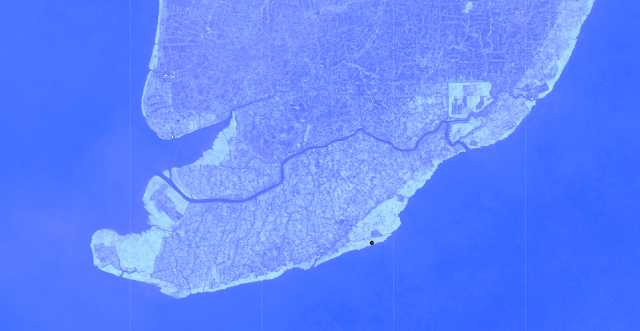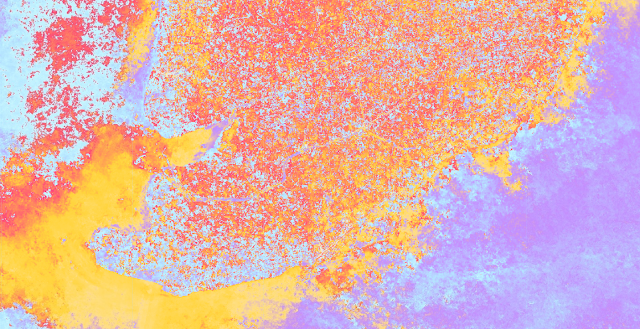## Tìm kiếm

The harmonic function (HF) itself is not easy for most of us to apply. In image processing, there are only a few applications which support this function.

In the field of remote sensing/image processing, HF has been applied as a multi-dimensional key for image classification. It is really cool to use HF for a particular crop detection using time-series data. There are a number of studies using HF for crop detection or land use/land cover mapping (Wilson, 2018; Ghazaryan, 2018Fawcett, 2017; Shew, 2017; Almeida, 2017; etc.). Most of them do not only use HF, but also apply decision tree or rule based algorithms for better outputs.

Some basic requirements for using HF in image processing include:

• a set of time-series satellite data;
• a set of samplings for the software to study;
• a set of rules to detect the object of interest;
• a set of auxilliary parameters (e.g. environmental condition, crop phenology...) to limit miss-interpretation; and finally,
• a good software/tool to implement.

This post demonstrates how to use Google Earth Engine to detect and observe Mangrove forest in southern Ca Mau province, Vietnam, using Landsat-8 time-series data.

The basic rule to detect mangrove using HF includes:

• To extract 3 important parameters: Phase, Magnitude and Value;
• To use more paramters for noise removal and miss-interpretation. They are: Standard deviation of NDVI; Mean of Water index.

Normally, Phase, Magnitude and Value are fine to detect mangrove. However, this type of plant is also similar to trees or forest in mainland. The use of Standard deviation of NDVI is good to detect trees with less change by season, such as mangrove. To improve accuracy, Water index can be used to extract only trees/forest above the water surface. In this case, it is quite suitable with mangrove forest as its living environment is in water or submerged areas.

Back to Google Earth Engine and the harmonic function, following codes demonstrate how to make use of Landsat time-series data and built-in harmonic function with GEE to extract Phase, Magnitude and Value.

//===============================================================
var date1 = '2017-01-01';
var date2 = '2018-12-31';
var roi = /* color: #d63000 */ee.Geometry.Point([105.08786, 8.6364]);
//===============================================================
// Load a collection of Landsat TOA reflectance images.
var landsatCollection = ee.ImageCollection('LANDSAT/LC08/C01/T1_TOA');
landsatCollection = landsatCollection.filterDate(date1, date2);
// The dependent variable we are modeling.
var dependent = 'NDVI';
// The number of cycles per year to model.
var harmonics = 1;
// Make a list of harmonic frequencies to model.
// These also serve as band name suffixes.
var harmonicFrequencies = ee.List.sequence(1, harmonics);
// Function to get a sequence of band names for harmonic terms.
var constructBandNames = function(base, list) {
return ee.List(list).map(function(i) {
return ee.String(base).cat(ee.Number(i).int());
});
};
// Construct lists of names for the harmonic terms.
var cosNames = constructBandNames('cos_', harmonicFrequencies);
var sinNames = constructBandNames('sin_', harmonicFrequencies);
// Independent variables.
var independents = ee.List(['constant', 't'])
.cat(cosNames).cat(sinNames);
// Function to mask clouds in Landsat 8 imagery.
var score = ee.Algorithms.Landsat.simpleCloudScore(image).select('cloud');
};
// Function to add an NDVI band, the dependent variable.
return image
.rename('NDVI'))
.float();
};
// Function to add an NDWI band, the dependent variable.
return image
.rename('NDWI'))
.float();
};

// Function to add a time band.
// Compute time in fractional years since the epoch.
var years = image.date().difference('1970-01-01', 'year');
var timeRadians = ee.Image(years.multiply(2 * Math.PI)).rename('t');
var constant = ee.Image(1);
};
// Function to compute the specified number of harmonics
// and add them as bands.  Assumes the time band is present.
return function(image) {
// Make an image of frequencies.
var frequencies = ee.Image.constant(freqs);
// This band should represent time in radians.
var time = ee.Image(image).select('t');
// Get the cosine terms.
var cosines = time.multiply(frequencies).cos().rename(cosNames);
// Get the sin terms.
var sines = time.multiply(frequencies).sin().rename(sinNames);
};
};
var harmonicLandsat = landsatCollection
//.filterBounds(Mekong)
// The output of the regression reduction is a 4x1 array image.
var harmonicTrend = harmonicLandsat
.reduce(ee.Reducer.linearRegression(independents.length(), 1));
// Turn the array image into a multi-band image of coefficients.
var harmonicTrendCoefficients = harmonicTrend.select('coefficients')
.arrayProject()
.arrayFlatten([independents]);
// Compute fitted values.
var fittedHarmonic = harmonicLandsat.map(function(image) {
image.select(independents)
.multiply(harmonicTrendCoefficients)
.reduce('sum')
.rename('fitted'));
});
// Plot the fitted model and the original data at the ROI.
print(ui.Chart.image.series(fittedHarmonic.select(['fitted','NDVI']), roi, ee.Reducer.mean(), 30)
.setOptions({
title: 'Harmonic model: original and fitted values',
lineWidth: 1,
pointSize: 3,
}));

// Pull out the three bands we're going to visualize.
var sin = harmonicTrendCoefficients.select('sin_1');
var cos = harmonicTrendCoefficients.select('cos_1');
// Do some math to turn the first-order Fourier model into
// hue, saturation, and value in the range[0,1].
var magnitude = cos.hypot(sin).multiply(10);
var phase = sin.atan2(cos).unitScale(-Math.PI, Math.PI);
var val = harmonicLandsat.select('NDVI').reduce('mean');
var mean_NDWI =  harmonicLandsat.select('NDWI').reduce('mean');
var mean_pix = harmonicLandsat.select('NDVI').reduce('mean');
var stdNDVI = harmonicLandsat.select('NDVI').reduce(ee.Reducer.stdDev());
var landSat = landsatCollection.reduce('median');
// Turn the HSV data into an RGB image and add it to the map.
var seasonality = ee.Image.cat(phase, magnitude, val).hsvToRgb();

//Center the map
Map.centerObject(roi, 11);
//Original Landsat-8 composite
var vizParams = {bands: ['B5_median', 'B4_median', 'B3_median'], min: 0, max: 0.4};
//Water index NDWI
Map.addLayer(mean_NDWI,{palette: '#b6fffa,#95b8ff,#6d84ff,#4270ff,#093aff', min: -0.8, max: 0.8},"NDWI",false);
//Standard deviation of NDVI
//Seasonality
//Sampling point
//Phase
Map.addLayer(phase,{palette: '#caf8ff,#b4e5ff,#c48dff,#ffdf87,#ffd43c,#ff427f', min: 0, max: 1},'Phase',false);
//Magnitude
//Value of NDVI
Map.addLayer(val,{palette: '#f3f3f3,#ffdca8,#9abcff,#c2ffb6,#b7ff73,#57ff52,#63e71c,#38b80b', min: 0, max: 1},"NDVI",false);

Some snapshots of derivative parameters:Harmonic function of NDVIFalse Color Composite Landsat-8 (B5, B4, B3)NDVIMagnitudeNDWIPhaseStandard Deviation of NDVI

Based on those parameters, you can start working on algorithm to detect mangrove forest or any other crops. It's really full of fun playing with GEE and multiple criteria spatial analysis. I hope this post is useful to you.

Note: The layer "Mangrove 2000" was prepared using Landsat satellite data from the year 2000. More than 1,000 Landsat scenes obtained from the USGS Earth Resources Observation and Science Center (EROS) were classified using hybrid supervised and unsupervised digital image classification techniques. This database is the first, most comprehensive mangrove assessment of the world (Giri et al., 2011). Partial funding of this research was provided by NASA.

#### Xem thêm

1.2.